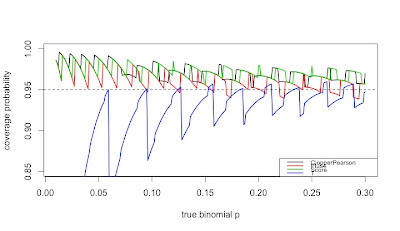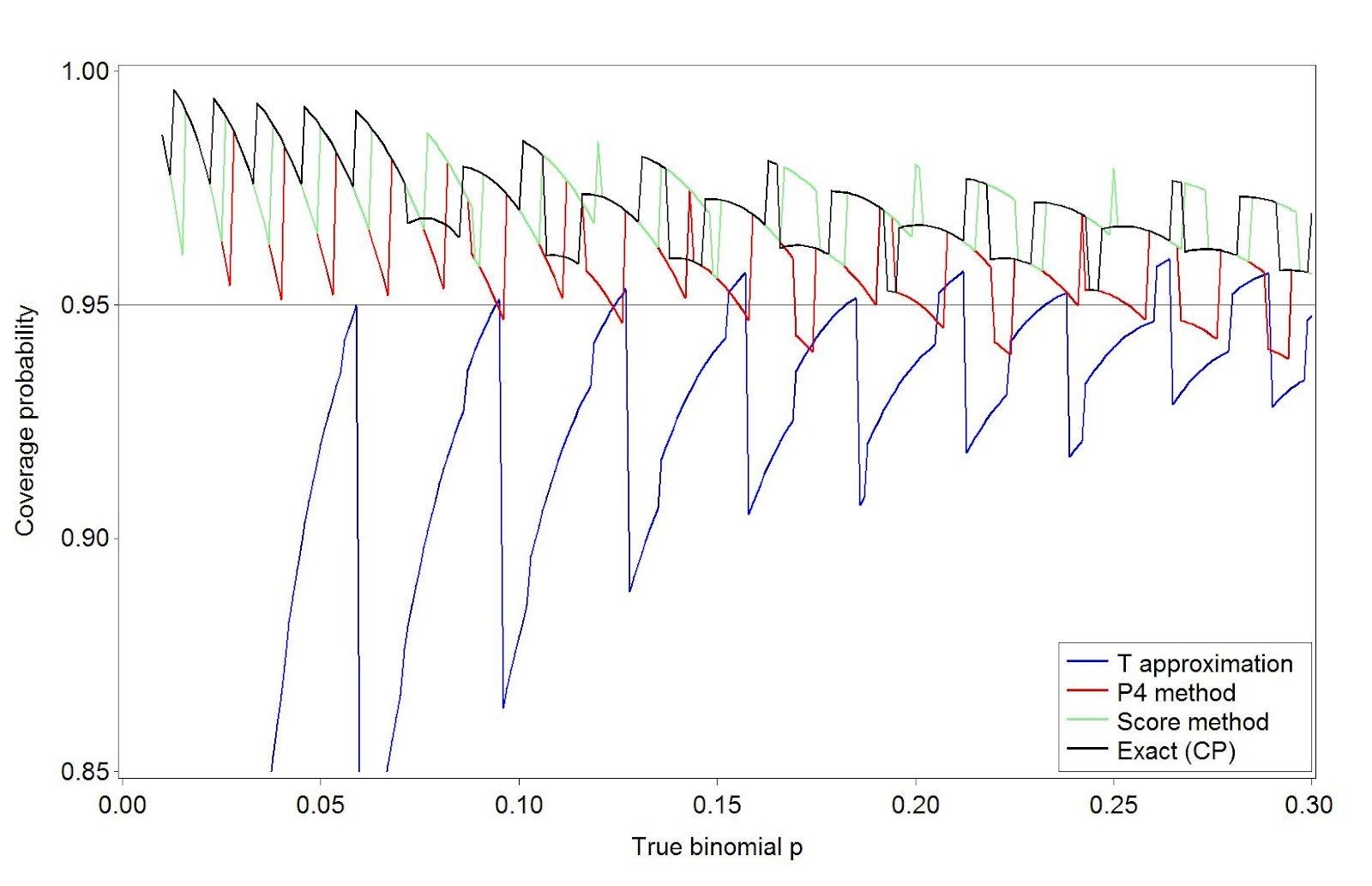## Monday, July 9, 2012

### Example 9.37: (Mis)behavior of binomial confidence intervalsWhile traditional statistics courses teach students to calculate intervals and test for binomial proportions using a normal or t approximation, this method does not always work well. Agresti and Coull ("Approximate is better than "exact' for interval estimation of binomial proportions". The American Statistician, 1998; 52:119-126) demonstrated this and reintroduced an improved (Plus4) estimator originally due to Wilson (1927).

In this entry, we demonstrate how the coverage varies as a function of the underlying probability and compare four intervals: (1) t interval, (2) Clopper-Pearson, (3) Plus4 (Wilson/Agresti/Coull) and (4) Score, using code contributed by Albyn Jones from Reed College. Here, coverage probability is defined as the expected value that a CI based on an observed value will cover the true binomial parameter that generated that value. The code calculates the coverage probability as a function of a given binomial probability p and a sample size n. Intervals are created for each of the possible outcomes from 0, ..., n, then checked to see if the intervals include the true value. Finally, the sum of the probabilities of observing outcomes in which the binomial parameter is included in the interval determines the exact coverage. Note several distinct probabilities: (1) binomial parameter "p", the probability of success on a trial; (2) probability of observing x successes in N trials, P(X=x); (3) coverage probability as defined above. For distribution quantiles and probabilities, see section 1.10 and table 1.1.

R

We begin by defining the support functions which will be used to calculate the coverage probabilities for a specific probability and sample size.
`CICoverage = function(n, p, alpha=.05) {  # set up a table to hold the results  Cover = matrix(0, nrow=n+1, ncol=5)  colnames(Cover)=c("X","ClopperPearson","Plus4","Score","T")  Cover[,1]=0:n  zq = qnorm(1-alpha/2)  tq = qt(1-alpha/2,n-1)  for (i in 0:n) {    Phat = i/n    P4 = (i+2)/(n+4)    # Calculate T and plus4 intervals manually,     # use canned functions for the other     TInt = Phat + c(-1,1)*tq*sqrt(Phat*(1-Phat)/n)    P4Int = P4 + c(-1,1)*zq*sqrt(P4*(1-P4)/(n+4))    CPInt= binom.test(i,n)\$conf.int    SInt = prop.test(i,n)\$conf.int    # check to see if the binomial p is in each CI     Cover[i+1,2] = InInt(p, CPInt)    Cover[i+1,3] = InInt(p, P4Int)    Cover[i+1,4] = InInt(p, SInt)    Cover[i+1,5] = InInt(p, TInt)  }  # probability that X=x   p = dbinom(0:n, n, p)  ProbCover=rep(0, 4)  names(ProbCover) = c("ClopperPearson", "Plus4", "Score", "T")  # sum P(X=x) * I(p in CI from x)  for (i in 1:4){    ProbCover[i] = sum(p*Cover[,i+1])  }  list(n=n, p=p, Cover=Cover, PC=ProbCover)}`

In addition, we define a function to determine whether something is in the interval.
`InInt = function(p,interval){  interval <= p && interval >= p}`

Finally, there's a function which summarizes the results.
`CISummary = function(n, p) {  M = matrix(0,nrow=length(n)*length(p),ncol=6)  colnames(M) = c("n","p","ClopperPearson","Plus4","Score","T")  k=0  for (N in n) {    for (P in p) {      k=k+1      M[k,]=c(N, P, CICoverage(N, P)\$PC)    }  }  data.frame(M)}`

We then generate the CI coverage plot provided at the start of the entry, which uses sample size n=50 across a variety of probabilities.
`lwdval = 2nvals = 50probvals = seq(.01, .30, by=.001)results = CISummary(nvals, probvals)plot(range(probvals), c(0.85, 1), type="n", xlab="true binomial p",     ylab="coverage probability")abline(h=0.95, lty=2)lines(results\$p, results\$ClopperPearson, col=1, lwd=lwdval)lines(results\$p, results\$Plus4, col=2, lwd=lwdval)lines(results\$p, results\$Score, col=3, lwd=lwdval)lines(results\$p, results\$T, col=4, lwd=lwdval)tests = c("ClopperPearson", "Plus4", "Score", "T")legend("bottomright", legend=tests,       col=1:4, lwd=lwdval, cex=0.70)`

The resulting plot is quite interesting, and demonstrates how non-linear the coverage is for these methods, and how the t (almost equivalent to the normal, in this case) is anti-conservative in many cases. It also confirms the results of Agresti and Coull, who concluded that for interval estimation of a proportion, coverage probabilities from inverting the standard binomial and too small when inverting the Wald large-sample normal test, with the Plus 4 yielding coverage probabilities close to the desired, even for very small sample sizes.

SAS
Calculating the coverage probability for a given N and binomial p can be done in a single data step, summing the probability-weighted coverage indicators over the realized values of the random variate. Once this machinery is developed, we can call it repeatedly, using a macro, to find the results for different binomial p. We comment on the code internally.
`%macro onep(n=,p=,alpha=.05);data onep;n = &n;p = &p;alpha = α/* set up collectors of the weighted coverage indicators */expcontrib_t = 0;expcontrib_p4 = 0;expcontrib_s = 0;expcontrib_cp = 0;/* loop through the possible observed successes x*/do x = 0 to n;  probobs = pdf('BINOM',x,p,n);  /* probability X=x */  phat = x/n;  zquant = quantile('NORMAl', 1 - alpha/2, 0, 1);  p4 = (x+2)/(n + 4);  /* calculate the half-width of the t and plus4 intervals */  thalf = quantile('T', 1 - alpha/2,(n-1)) * sqrt(phat*(1-phat)/n);  p4half = zquant * sqrt(p4*(1-p4)/(n+4));  /* the score CI in R uses a Yates correction by default, and is      reproduced here */  yates = min(0.5, abs(x - (n*p)));  z22n = (zquant**2)/(2*n);  yl = phat-yates/n;  yu = phat+yates/n;  slower = (yl + z22n - zquant * sqrt( (yl*(1-yl)/n) + z22n / (2*n) )) /    (1 + 2 * z22n);   supper = (yu + z22n + zquant * sqrt( (yu*(1-yu)/n) + z22n / (2*n) )) /    (1 + 2 * z22n);   /* cover = 1 if in the CI, 0 else */  cover_t = ((phat - thalf) < p) and ((phat + thalf) > p);  cover_p4 = ((p4 - p4half) < p) and ((p4 + p4half) > p);  cover_s = (slower < p) and (supper > p);  /* the Clopper-Pearson interval can be easily calculated on the fly */   cover_cp = (quantile('BETA', alpha/2 ,x,n-x+1) < p) and     (quantile('BETA', 1 - alpha/2 ,x+1,n-x) > p);   /* cumulate the weighted probabilities */  expcontrib_t = expcontrib_t + probobs * cover_t;  expcontrib_p4 = expcontrib_p4 + probobs * cover_p4;  expcontrib_s = expcontrib_s + probobs * cover_s;  expcontrib_cp = expcontrib_cp + probobs * cover_cp;  /* only save the last interation */  if x = N then output;  end;run;%mend onep;`

The following macro calls the first one for a series of binomial p for a fixed N. Since the macro %do loop can only iterate through integers, we have to do a little division; the %sysevelf function will do this within the macro.
`%macro repcicov(n=, lop=, hip=, byp=, alpha= .05);/* need an empty data set to store the results */data summ; set _null_; run;%do stepp = %sysevalf(&lop / &byp, floor) %to %sysevalf(&hip / &byp,floor);  /* note that the p sent to the %onep macro is a                            text string like "49 * .001" */  %onep(n = &n, p = &stepp * &byp, alpha = &alpha);  /* tack on the current results to the ones finished so far */  /* this is a simple but inefficient way to add each binomial p into      the output data set */  data summ; set summ onep; run;%end;%mend repcicov;/* same parameters as in R */%repcicov(n=50, lop = .01, hip = .3, byp = .001);`

Finally, we can plot the results. One option shown here and not mentioned in the book are the mode=include option to the symbol statement, which allows the two distinct pieces of the T coverage to display correctly.
`goptions reset=all;legend1 label=none position=(bottom right inside)        mode=share across=1 frame value = (h=2);axis1 order = (0.85 to 1 by 0.05) minor=none    label = (a=90 h=2 "Coverage probability") value=(h=2);axis2 order = (0 to 0.3 by 0.05) minor=none    label = (h=2 "True binomial p") value=(h=2);symbol1 i = j v = none l =1 w=3 c=blue mode=include;symbol2 i = j v = none l =1 w=3 c=red;symbol3 i = j v = none l =1 w=3 c=lightgreen;symbol4 i = j v = none l =1 w=3 c=black;proc gplot data = summ;plot (expcontrib_t expcontrib_p4  expcontrib_s  expcontrib_cp) * p   / overlay legend vaxis = axis1 haxis = axis2 vref = 0.95 legend = legend1;label expcontrib_t = "T approximation" expcontrib_p4 = "P4 method"      expcontrib_s = "Score method" expcontrib_cp = "Exact (CP)";run; quit;`Paul Hewson said...

Hi Nick. Very nice blog post. But do you have any thoughts on why we still teach Normal approximations to the Binomial these days? I'm not sure we need to.

Nick Horton said...

I don't actually teach normal approximations to the binomial, which is why I was struck by the figure when Albyn pointed this out to me. I would agree that this probably isn't a key concept to communicate in intro stats.

Ken Kleinman said...

I assume it's still taught in some places due to inertia. But when included with due consideration, one reason might be to demonstrate an application of the normal approximation. Which is, in fact, remarkably good in many cases.

Ken said...

Would be interesting to see the parametric and nonparametric bootstrap, and why not the Bayesian as well.

Derrick said...

I would like to add the coverage properties of cassella's exact method which is am improvement of the cp interval for comparison reasons. It is implemented in statexact. How would I do that with the sas macro? Thanks

Alfred Lim said...

Hi, can I know why when I generate this SAS code, I can get the graph, but there is no lines drawn on it. Which code got problem?

Ken Kleinman said...

@Derrick-- I think I'd generate the data in StatXact and then read it into SAS and merge it into the final data set after running the macro. Mayeb if you had proc statxact you could do it differently?

@Alfred: When I just ran it, it worked fine for me, excecpt that Blogger turned α into a greek alpha with no semicolon. Did you cope/paste from the blog, or try to re-tyoe all of this?

Alfred Lim said...

@Ken : Hi, I copy and paste. No matter I use a or alpha greek. There is still no lines drawn in the graph. I guess there is something wrong in the code?

Ken Kleinman said...

Very strange. I copied and pasted also, and it worked for me.

Alfred Lim said...

@Ken nvm, I use R programming and can get the result already. Thanks.

Alfred Lim said...
This comment has been removed by the author.
Alfred Lim said...
This comment has been removed by the author.
Alfred Lim said...

Hi, I want to find the coverage probabilities of 2 different binomial proportions, for example fixing Phat2 = 0.1 and n1=n2=20. The formula is
TInt = (Phat1 - Phat2) + c(-1,1)*tq*sqrt(Phat1*(1-Phat1)/n1 + Phat2*(1-Phat2)/n2)
I did some modifications but still get a weird result, anyone willing to help me?
I wonder maybe I got wrong in this code
p = dbinom(0:m, m, p), perhaps for proportion difference, different code will be used?

Hydra Viridis said...

Hi, very nice and useful.
I admit I was not aware about such misbehavior. During last days at an interim analysis (not planned) I found a greater power with less patients as required by the final analysis ...you know I was just a bit frightened it was my fault. :-)